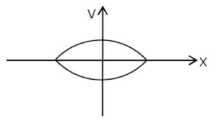# When a particle executes SHM, the nature of graphical representation of velocity as a function of displacement is :

Question:

When a particle executes SHM, the nature of graphical representation of velocity as a function of displacement is :

1. (1) elliptical

2. (2) parabolic

3. (3) straight line

4. (4) circular

Correct Option: 1

Solution:

(1)

We know that is SHM:

$V=\omega \sqrt{A^{2}-x^{2}}$